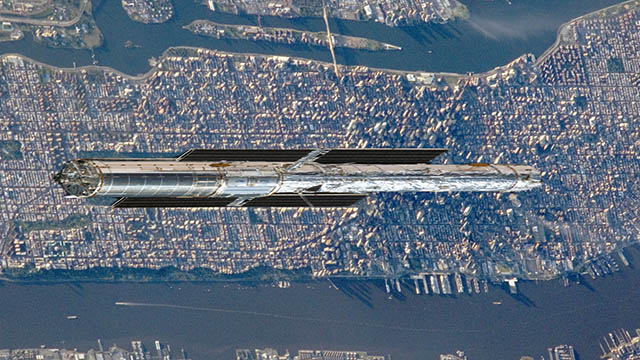# View an Apollo flag on the Moon from Earth?

How can we see one of the flags left on the Moon by the Apollo missions? Let’s start with a telescope that can already do this, the Lunar Reconnaissance Orbiter Camera (LROC). The image below of the Apollo 16 landing site is just barely able to resolve the flag and its shadow.LROC. M175179080L [NASA/GSFC/Arizona State University]

The LRO is a spacecraft in orbit around the moon and close enough (about 50 km) to capture these images with a small telescopic camera.

The pixel pitch of this LROC image is 0.25 meters per pixel. This corresponds to an optical resolution 0f 0.5 meters by the sampling theorem. What would it take to capture this image from the Earth?

### Telescope aperture

The resolution of any telescope is limited by diffraction. One measure of this is the Rayleigh Criterion which gives the angular resolution of a telescope in terms of its aperture D and the wavelength of the light wl:

ar = 1.22 wl / D

solving for D

D = 1.22 wl / ar

Because the angle is very small the resolution angle r in radians is just the resolution distance on the moon divided by the distance to the moon:

ar = 0.25 / 3.84x10^8 = 6.5x10^(-10)

The sampling theorem requires an optical resolution of twice this distance: 1.3x10^(-9).

For visible light, let’s choose the wavelength of green light as wl, about 5.5x10^(-7) meters, because the moon may be made of green cheese.

D = 1.22 * 5.5x10^(-7) / 1.3x10^(-9)
D = 516 m

The telescope will need an objective aperture of just over one half kilometer.

### Telescope focal length

We also need the telescope focal length required to get enough magnification to capture this image. Typical camera sensor pixel pitches range from 2 to 8 microns. Choosing 4 microns as our pixel pitch.

The plate scale, ps, of an image at a telescope’s prime focus is the angular size of the image, a, divided by its linear size, s, at the focal plane. This is inversely proportional to the focal length, fl.

ps = a / s = 1 / fl

The required image scale for an image equivalent to the LROC image is 0.25 m per pixel.

fl = s / a

fl = 4 x 10^(-6) / 6.5x10^(-10) = 6.7x10^3 meters = 6.7 kilometers

In order to avoid blurring from atmospheric turbulence the telescope would need to be in low earth orbit.

### A Moon flag telescope imagined

Here is an image of how this telescope size compares to midtown Manhattan. The diameter of the telescope would span nearly 2/3 of the width of Central Park and the focal length would reach from the northern edge of Central Park past Madison Square Garden.NASA image of Manhattan from the ISS and stretched HST image composite

Content created: 2019-07-21